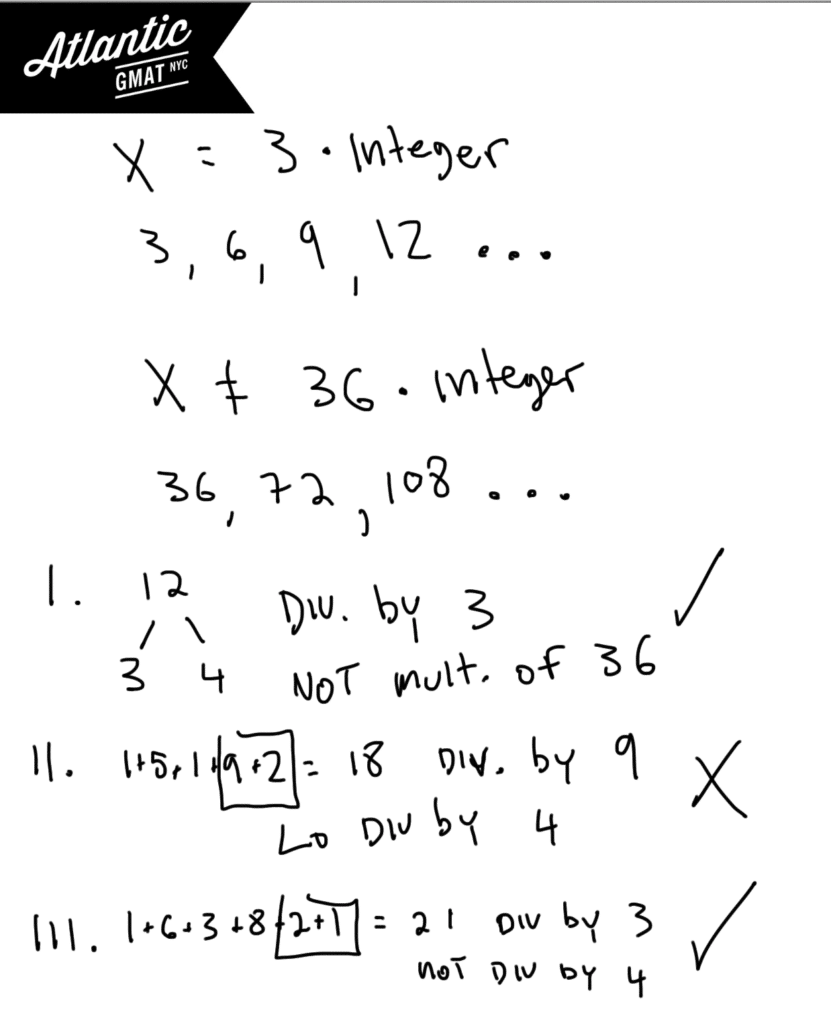# GMAT Problem Solving

GMAT Problem solving should be familiar: 5 choice multiple choice. There's nothing specifically GMAT about the format except that it's computer adaptive. The content on the Problem Solving is the same as that on the Data Sufficiency (DS). The Problem Solving differs from the DS in that you do need to solve for an answer and hence need to do more arithmetic than is necessary on the Data Sufficiency.

## GMAT Problem Solving Example

If x is a positive multiple of 3 but not divisible by 36 then which of the following values could be a factor of x?

I. 12

II. 15192

III. 163821

A. I Only

B. II Only

C. III Only

D. I and III Only

E. I, II, and III

I spend a lot time in GMAT tutoring sessions teaching how to recognize GMAT question types. By recognizing the question you can apply the right tools to get to the answer. When GMAT questions have such keywords as divisible by, multiple of, and factor of you should be thinking about divisibility and prime factors.  In this question we have a few of these words and so should be considering the prime factors (or the very least the factors) of these numbers. We know that x is a positive multiple of 3 so that x could be 3, 6, 9... We also know that x is not a multiple of 36. So that x cannot have 9 and 4 as factors. In this case we don't need to worry about the complete prime factorization because it's easy enough to see what is happening. That means that x can be any multiple of 3 that is not divisible by both 9 and 4. Another way to say that is that x can't be a number with two 2's and 3's. x can have to two 2's but not two 3's or two 3's but not two 2's. We just can't have both of those numbers together because then the number will be divisible by 36 - a situation that is out of bounds in this question.

In the answer choices you need to eliminate answers that are either

1. Not divisible by 3

2. Divisible by both 4 and 9 (divisible by 36)

In this question and in many others you will be helped by knowing some basic divisibility rules. Any number whose digits add to 3 are divisibly by 3. Any number whose digits add to 9 is divisible by 9. If the last two digits of a number form a number that is divisible by 4 then the entire number is divisible by 4. You can use these rules to eliminate choice II. It is divisibly by both 9 and 4. Choices I and III both follow our rules. The answer is D.## How to study for GMAT Problem Solving?

-Same as with Data Sufficiency: Focus on set-up. Avoid running headfirst into the calculations. This is a critical thinking test not a math test. Make sure that you are taking the time to read and process.

-After you've defined the question and are ready to apply the details, again, take your time. The biggest drain I see on our GMAT tutoring students' scores is questions lost by careless calculations. Dotting your I's and crossing your T's will give you a GMAT score boost without learning new content.

-Yes there are specific GMAT puzzles to be mastered BUT focus on the fundamentals. Make sure you've got all of the basics backwards and forwards before getting into more esoteric GMAT content. You could probably break 700 knowing nothing about combinatorics and probability. That's not to say that you shouldn't have those things covered but just that you shouldn't obsess about specific content before mastering GMAT fundamental quant.

# CONTACT

Atlantic GMAT

405 East 51st St.

NY, NY 10022

(347) 669-3545

info@AtlanticGMAT.com